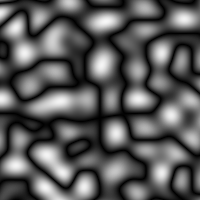# noiseField

field
noiseField
RayTK
v0.36

A float or vector field that uses one of several noise functions.

Some of these may be costly to compute, so pay attention to frame rate when using them.

The different types of noise use different types of coordinates. If the type of coordinate used by `Noisetype` doesn’t match the `Coordtype`, any missing parts will be replaced with zeros. When the `Coordtype` is 3D but the `Noisetype` only uses 2D, the `Axis` parameter determines which parts of the coordinates are used.

For types that use 4D coordinates, the `Translate` and `Scale` parameters can still be used to control the 4th coordinate.

## Parameters

• `Noise Type`: The type of noise function.
• `Simplex 2D (TD)`:
• `Simplex 3D (TD)`:
• `Simplex 4D (TD)`:
• `Perlin 2D (TD)`:
• `Perlin 3D (TD)`:
• `Perlin 4D (TD)`:
• `Classic Perlin 2D (glslNoise)`:
• `Classic Perlin 3D (glslNoise)`:
• `Classic Perlin 4D (glslNoise)`:
• `Simplex 2D (glslNoise)`:
• `Simplex 3D (glslNoise)`:
• `Simplex 4D (glslNoise)`:
• `Cheap Random Lookup (TD)`:
• `Cellular 2D (wombat)`:
• `Cellular 2D Deriv (wombat)`:
• `Cellular 3D (wombat)`:
• `Cellular 3D Deriv (wombat)`:
• `Value 2D (wombat)`:
• `Value 2D Deriv (wombat)`:
• `Value 3D (wombat)`:
• `Value 3D Deriv (wombat)`:
• `Value 4D (wombat)`:
• `Coord Type`: The type of coordinates that the op supports.
• `Auto`:
• `1D`:
• `2D`:
• `3D`:
• :
• `Axis`: When the `Noisetype` uses 2D coordinates but `Coordtype` is 3D, this is used to choose which plane of the coordinates are used.
• `X`: Use Y and Z.
• `Y`: Use Z and X.
• `Z`: Use X and Y.
• `Translate`: Offsets the coordinates used to calculate noise.
• `Scale`: Scales the coordinates used to calculate noise.
• `Amplitude`: Multiplies the amount produced by the noise.
• `Offset`: Offsets (adds to) the amount produced by the noise.

## Inputs

• `Coordinate Field`: (optional)
• Coordinate Types: `float` `vec2` `vec3` `vec4`
• Context Types: `Context` `MaterialContext` `CameraContext` `LightContext` `RayContext` `ParticleContext`
• Return Types: `float` `vec4`